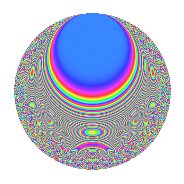# Properties

 Label 4031.2.a.dLevel 4031 Weight 2 Character orbit 4031.a Self dual Yes Analytic conductor 32.188 Analytic rank 0 Dimension 98 CM No

# Related objects

## Newspace parameters

 Level: $$N$$ = $$4031 = 29 \cdot 139$$ Weight: $$k$$ = $$2$$ Character orbit: $$[\chi]$$ = 4031.a (trivial)

## Newform invariants

 Self dual: Yes Analytic conductor: $$32.1876970548$$ Analytic rank: $$0$$ Dimension: $$98$$ Fricke sign: $$-1$$ Sato-Tate group: $\mathrm{SU}(2)$

## $q$-expansion

The dimension is sufficiently large that we do not compute an algebraic $$q$$-expansion, but we have computed the trace expansion.

 $$\operatorname{Tr}(f)(q) =$$ $$98q + 6q^{2} + 6q^{3} + 116q^{4} + q^{5} + 7q^{6} + 12q^{7} + 15q^{8} + 136q^{9} + O(q^{10})$$ $$\operatorname{Tr}(f)(q) =$$ $$98q + 6q^{2} + 6q^{3} + 116q^{4} + q^{5} + 7q^{6} + 12q^{7} + 15q^{8} + 136q^{9} + 16q^{10} + 25q^{11} + 8q^{12} + 18q^{13} + 34q^{14} + 14q^{15} + 136q^{16} + 35q^{17} + 20q^{18} + 48q^{19} - 20q^{20} + 62q^{21} + 32q^{22} + q^{23} + 8q^{24} + 173q^{25} + 15q^{26} + 18q^{27} + 37q^{28} + 98q^{29} - 6q^{30} + 20q^{31} + 16q^{32} + 24q^{33} - 6q^{34} + 11q^{35} + 155q^{36} + 62q^{37} - 5q^{38} + 45q^{39} + 38q^{40} + 50q^{41} + 7q^{42} + 72q^{43} + 92q^{44} - 13q^{45} + 32q^{46} - 16q^{47} - 13q^{48} + 194q^{49} + 50q^{50} + 2q^{51} + 83q^{52} - 11q^{53} + 58q^{54} + 32q^{55} + 106q^{56} + 84q^{57} + 6q^{58} + 20q^{59} + 62q^{60} + 142q^{61} - 27q^{62} + 26q^{63} + 213q^{64} + 13q^{65} - 35q^{66} + 5q^{67} + 69q^{68} + 68q^{69} - 2q^{70} - 10q^{71} - 9q^{72} + 74q^{73} + 21q^{74} + 19q^{75} + 116q^{76} + 41q^{77} - 162q^{78} + 104q^{79} - 86q^{80} + 230q^{81} + 53q^{82} - 19q^{83} + 76q^{84} + 125q^{85} + 9q^{86} + 6q^{87} + 68q^{88} + 120q^{89} + 122q^{90} + 30q^{91} + 3q^{92} - 56q^{93} + 22q^{94} + 32q^{95} - 55q^{96} + 98q^{97} - 15q^{98} + 69q^{99} + O(q^{100})$$

## Embeddings

For each embedding $$\iota_m$$ of the coefficient field, the values $$\iota_m(a_n)$$ are shown below.

For more information on an embedded modular form you can click on its label.

Label $$a_{2}$$ $$a_{3}$$ $$a_{4}$$ $$a_{5}$$ $$a_{6}$$ $$a_{7}$$ $$a_{8}$$ $$a_{9}$$ $$a_{10}$$
1.1 −2.78351 2.95190 5.74795 −3.19044 −8.21665 −4.40114 −10.4325 5.71371 8.88064
1.2 −2.76750 0.603367 5.65907 3.54828 −1.66982 1.63115 −10.1265 −2.63595 −9.81988
1.3 −2.75511 −2.40315 5.59062 −0.846757 6.62092 3.63959 −9.89254 2.77511 2.33291
1.4 −2.74332 −0.716173 5.52580 −3.18087 1.96469 1.27957 −9.67240 −2.48710 8.72614
1.5 −2.61634 −3.34235 4.84525 −3.10216 8.74473 −4.97241 −7.44414 8.17130 8.11632
1.6 −2.59643 0.964956 4.74146 3.18498 −2.50544 −0.304804 −7.11802 −2.06886 −8.26958
1.7 −2.58392 3.19893 4.67665 1.67511 −8.26577 1.22424 −6.91625 7.23312 −4.32836
1.8 −2.57508 1.48970 4.63103 −2.75202 −3.83609 2.46323 −6.77510 −0.780799 7.08668
1.9 −2.48847 −3.00036 4.19251 0.297405 7.46633 −2.60645 −5.45599 6.00219 −0.740084
1.10 −2.44621 0.244458 3.98396 −3.48270 −0.597997 1.44143 −4.85320 −2.94024 8.51943
1.11 −2.43943 −2.23361 3.95082 1.05551 5.44873 −1.84487 −4.75890 1.98900 −2.57484
1.12 −2.22708 1.27662 2.95990 0.197788 −2.84313 −3.89763 −2.13778 −1.37025 −0.440491
1.13 −2.11438 2.42067 2.47061 1.02780 −5.11822 0.853130 −0.995051 2.85965 −2.17316
1.14 −2.08450 0.675318 2.34516 −1.05546 −1.40770 −4.46025 −0.719483 −2.54395 2.20012
1.15 −2.03151 −1.34287 2.12703 3.37426 2.72805 0.525143 −0.258068 −1.19670 −6.85484
1.16 −1.99223 −2.07417 1.96900 0.414014 4.13224 4.11338 0.0617619 1.30220 −0.824814
1.17 −1.97068 0.422540 1.88358 2.63171 −0.832693 −1.79791 0.229417 −2.82146 −5.18627
1.18 −1.96419 −0.913998 1.85806 −4.29424 1.79527 −2.01487 0.278799 −2.16461 8.43472
1.19 −1.93664 0.437969 1.75056 3.14696 −0.848187 5.11562 0.483081 −2.80818 −6.09452
1.20 −1.86328 0.307270 1.47182 0.176749 −0.572530 −0.402020 0.984149 −2.90559 −0.329334
See all 98 embeddings
 $$n$$: e.g. 2-40 or 990-1000 Embeddings: e.g. 1-3 or 1.98 Significant digits: Format: Complex embeddings Normalized embeddings Satake parameters Satake angles

## Inner twists

This newform does not have CM; other inner twists have not been computed.

## Atkin-Lehner signs

$$p$$ Sign
$$29$$ $$-1$$
$$139$$ $$1$$

## Hecke kernels

This newform can be constructed as the kernel of the linear operator $$T_{2}^{98} - \cdots$$ acting on $$S_{2}^{\mathrm{new}}(\Gamma_0(4031))$$.